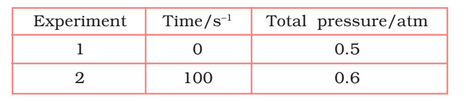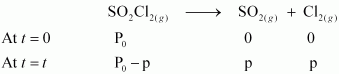# 4.21   The following data were obtained during the first order thermal decomposition of  at a constant volume.Calculate the rate of the reaction when total pressure is 0.65 atm.

M manish

The thermal decomposition of  is shown here;After t time, the total pressure

So,

thus,

for first order reaction,

now putting the values of pressures, when t = 100s

when

= 0.65 - 0.5
= 0.15 atm

So,
= 0.5 - 0.15
= 0.35 atm

Thus, rate of reaction, when the total pressure is 0.65 atm
rate = k()
=
= 7.8

Exams
Articles
Questions